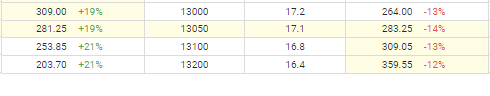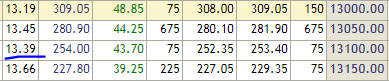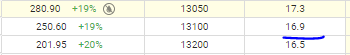# Does sensibull and Zerodha's BSM option price calculators use different formulas?

@Sensibull @siva @ShubhS9 how do i fairly price a option
For example:For the 13100CE December expiry the trading price is 253 rupees and when i input the same parameters and even with a lower implied volatility the price is still 10 points below. Since Implied volatility itself is derived from the price technically if the price is lower then the implied volatility should be lower too given all other things are assumed to be constant.

In Interest Rate colum, you can use RBI 91 Day Treasury Bill rate of 3%, which prices Options much closer to their CMP, the BSM shows you theoretical price so it won’t be 100% accurate, there will always be some variance.

ohkk, i understood the interest rate mistake. thankyou. My one last question is that i agree BSM model is theoretical but the implied volatility is practical right. It is derived from the price itself. So if i put the exact same values of implied volatility that is see on the sensibull option chain into the BSM Calculator then i should get the exact same answer right because the BSM formula is the same everywhere. Unless Sensibull Uses some weighted future’s price i guess to calculate their implied volatility.Also the Implied volatilities for the same expiry are very different in @Sensibull and the NSE Option Chain.

This has been explained by Sensibull here.

2 Likes